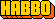- Habbo Development Wiki - Communication - Encoding

>TIP: This part might be easier to work with by making use of the Habbo Decoder tool.

FUSE 0.2.0 makes use of two number encoding methods:

• Base64: The first one, and the most used one throughout communications and easier to understand, makes use of ASCII characters in a Base64 format.
• VL64 (Wire): The second one combines the use of Base4 with Base64, also represented in ASCII.

Base64

Base64 is an encoding scheme which makes use of radix-64 values.

It works the same way as radix-10 numbers (0-9), but instead makes use of ASCII characters values to go from 0 to 63.

Its encoding length must be previously known, hence Base64-encoded numbers use @ (0 in Base4, 64 in ASCII due to its +64 offset) as padding.

Decoding

ASCII characters numeric values have an offset of +64, so in order to obtain the true value of a single byte, 64 must be substracted to it. The rest of it works the same way as radix-10 numbers do.

Java example:

public static int decode(char[] input) {
int output = 0;
int exp = input.length - 1;

for (int i = 0; i < input.length; i++) {
output += ((int)input[i] % 64) * (int)Math.pow(64, exp);
exp--;
}

return output;
}

Encoding

Opposite of encoding, 64 must be added to each byte to get its ASCII counterpart.

The following axample encodes from a given radix-10 number to Base64 in a fixed length of 2 bytes, using @ as padding.

Java example:

public static String encode(int input) {
String output = Character.toString((char)(input / 64 + 64)) + (char)(input % 64 + 64);
return output;
}

VL64 (Wire)

VL64, also known as Wire, is a variable length encoding scheme which makes use of a mix of radix-4 and radix-64 values.

Base64 numbers must be understood in order to comprehend VL64.

Obtaining length

Unlike Base64, VL64 length is not known in advance. The first byte is read to know the total size in bytes/characters of the encoded number, including this first byte in the length.

@ A B C 0 0 0 Chars Length Min value Max value

Even rows represent positive numbers and odd rows are negative, as indicated by the min and max values.

The following method gets first byte in the VL64 as parameter, substracts 64 from it to get obtain Base64 value and then divides by 8 to get its length, like indicated in the table above.

Java example:

public static int getLength(byte input) {
return (input - 64) / 8;
}

Decoding

The first byte is not only used to indicate length, but is also a Base4-encoded number being part of the total value, being the value of each number in the first column of the table above 0, 1, 2 and 3, respectively, also applying ASCII +64 offset.

However, at the time of calculating the total value of the encoded number, this character does not act at the front of the value as it might seem logical, but it gets moved to the end of the encoded number.

Java example:

public static int decode(byte[] input) {
int output = input & 3;
int length = (input - 64) / 8;

for (int i = 0; i < length; i++) {
output += ((int)input[i] - 64) * ((int)Math.pow(64, i) / 16);
}

return (input & 7) < 4 ? output : -output;
}

Encoding

Encoding process makes the opposite of decoding; the first Base4 number is calculated at the start, then followed by the Base64 numbers.

Finally, it gets cropped to its appropiate length depending on the number of calculated Base64 numbers.

Java example:

public static byte[] encode(int input) {
int posInput = Math.abs(input);
int length = 1;
byte[] output = new byte;

output = (byte)(posInput & 3 + (input >= 0 ? 64 : 68));
posInput /= 4;

for (int i = 1; i <= 5; i++) {
output[i] = (byte)(posInput % 64 + 64);
posInput /= 64;
if (output[i] != 64) length = i + 1;
}

output += (byte)(length * 8);
return new String(Arrays.copyOf(output, length)).getBytes();
}